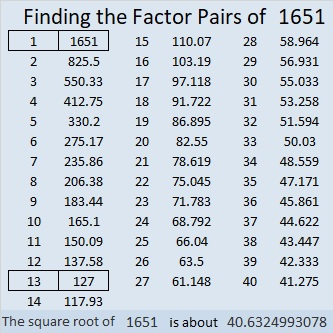# 1651 Multiplication Fun

Contents

### Today’s Puzzle:

Look how much fun these kids are having doing multiplication!

A game like that can help kids get ready to solve a fun puzzle based on the multiplication table.

Write each number from 1 to 10 in both the first column and the top row so that those numbers and the given clues become a multiplication table.### Factors of 1651:

• 1651 is a composite number.
• Prime factorization: 1651 = 13 × 127.
• 1651 has no exponents greater than 1 in its prime factorization, so √1651 cannot be simplified.
• The exponents in the prime factorization are 1 and 1. Adding one to each exponent and multiplying we get (1 + 1)(1 + 1) = 2 × 2 = 4. Therefore 1651 has exactly 4 factors.
• The factors of 1651 are outlined with their factor pair partners in the graphic below.### More About the Number 1651:

1651 is the hypotenuse of a Pythagorean triple:
635-1524-1651, which is (5-12-13) times 127.

1651 is the 26th heptagonal number because
5(26²)/2 – 3(26)/2 = 1651.

1651 is a nice-looking palindrome in base 2:
1651₁₀ = 11001110011₂.
That just means that
2¹⁰ + 2⁹ + 2⁶ + 2⁵ + 2⁴+ 2¹+ 2⁰ = 1024 + 512 + 64 + 32 + 16 + 2 + 1 = 1651.

This site uses Akismet to reduce spam. Learn how your comment data is processed.